# Farshad Oil Compressibility correlation

Jump to: navigation, search

## Farshad oil compressibility correlation

Farshad correlation is an empirical correlation for the oil compressibility published in 1996.

## Math & Physics

A1 = 0.1982
A2 = 0.6685
A3 = –0.21435
A4 = 1.0116
A5 = -0.1616
A6 = –5.4531
A7 = 5.03 * 1e-4
A8 = 3.5 * 1e-8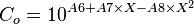$C_o = 10^{A6 + A7 \times X - A8 \times X^2}$

where: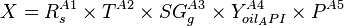$X = R^{A1}_s \times T^{A2} \times SG^{A3}_g \times Y^{A4}_{oil_API} \times P^{A5}$

## Nomenclature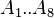$A_1..A_{8}$ = coefficients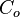$C_o$ = oil compressibility, psia-1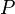$P$ = pressure, psia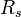$R_s$ = solution oil gas ratio, scf/bbl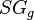$SG_g$ = gas specific gravity, dimensionless$T$ = temperature, °F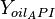$Y_{oil_API}$ = oil API gravity, °API